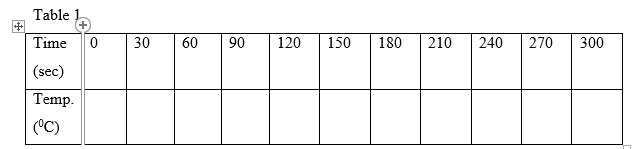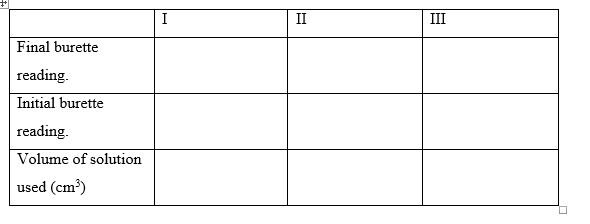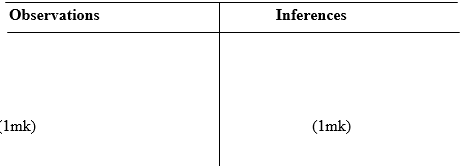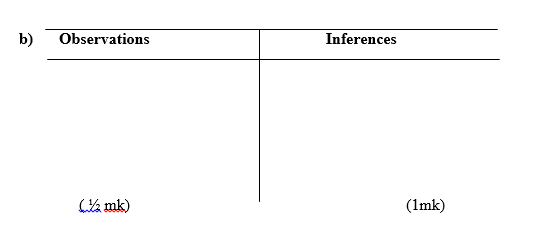##### Search### KCSE CLUSTER TESTS 27

#### Chemistry Paper 3

1.

You are provided with

• Magnesium ribbon, solid A.
• 0.7M Hydrochloric acid, solution B.
• 0.3M Sodium hydroxide, solution C.
• Distilled water.

You are required to determine the

: i) Temperature change when Magnesium reacts with excess hydrochloric acid

. ii) Number of moles of Hydrochloric acid that remain unreacted.

iii) Number of moles of Magnesium that reacted .

iv) Molar heat of reaction between Magnesium and Hydrochloric acid.

Procedure 1

• Using a burette, measure 50cm3 of solution B and place it in a100ml beaker. Measure the temperature of the solution B in the 100ml beaker and record the value in the table I
• Put the magnesium ribbon in the 50cm3 of solution B in the 100ml beaker immediately and start the stop watch. Stir the mixture continuously with the thermometer making sure the magnesium ribbon remains inside solution as it reacts.
• Measure the temperature after every 30 seconds and record the values in the table I. Continue stirring and measuring the temperature to complete table I
• Keep the resulting solution for procedure 2i) Plot a graph of temperature (y- axis) against time on the grid provided.

GRID

ii) On the graph show the maximum change in temperature ,∆T,and determine its value, ∆T. (1mk) Procedure 2

• Transfer all the solution obtained in procedure 1 into 250ml conical flask. Clean the burette and use it to place 50cm3 of distilled water into the beaker used in the procedure 1.
• Transfer all the 50cm3 of water into 250ml conical flask containing the solution from procedure 1. Label this solution as D.
• Empty the burette and fill it with solution C. Pipette 25cm3 of solution D and place it into an empty 250ml conical flask .Add two drops of phenolphthalein indicator and titrate solution C against solution D.
• Record the results in table 2 and complete the table 2.
•i Calculate the average volume of solution C used.

ii) Calculate the number of moles of: I) 0.3M sodium hydroxide used.

II) Hydrochloric acid in 25cm3 of solution D.

III) Hydrochloric acid in 100cm3of solution D.

IV) Hydrochloric acid in 50cm3 of solution B.

V) hydrochloric acid that reacted with magnesium

VI) Magnesium that reacted

c) Using your answer in (VI) above , determine the molar heat of reaction between Magnesium and Hydrochloric acid (assume the heat capacity of the solution is 4.2Jg-1K-1 and density is 1.0gcm-3.

15 marks

2.

You are provided with solid E. Carry out the following tests and write your observations and inferences in the spaces provided. a) Place about one half of solid E in a dry test-tube . Heat it strongly and test any gas produced using hydrochloric acid; solution B on a glass rodb) Place the rest of solid E in a boiling tube. Add about 10cm3 of distilled water. Shake well and use 2cm3 portions for each of the test below.i ii) To the second portion, add about 1cm3 of hydrochloric acid solution B.iii) To the third portion, add two drops of aqueous Lead (II) nitrate and heat the mixture to boiling.15 marks

3.

You are provided with solid P. Carry out the following test and record your observations and inferences in the spaces provided. a) Place about one half of solid P in a dry test-tube . Retain the other half of solid P for use in

(b). Add all the absolute ethanol provided to solid P in the test-tube . Shake the mixture.Divide the mixture into two portions

i) Determine the PH of the first portion using universal indicator solution and PH chart.ii) To the second portion, add one half of the solid Sodium hydrogen carbonate.b) Place the remaining amount of solid P in a boiling tube. Add 10cm3 of distilled water and shake. Boil the mixture and divide it into three portions while still warm.

i) To the first portion, add the remaining amount of solid Sodium Hydrogen carbonateii) To the second portion, add three drops of acidified potassium dichromate (VI) solution and warm.iii) To the third portion, add three drops of Bromine water.10 marks

Back Top